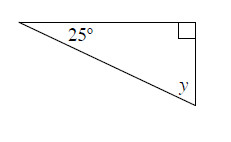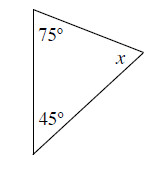### Home > MC1 > Chapter 8 > Lesson 8.3.2 > Problem8-94

8-94.

Find the measure of the missing angle in each triangle below.

1.Since we know that the angles of a triangle add together to $180^\circ$ and that the right angle is $90^\circ$, we know that $25^\circ + 90^\circ + y = 180^\circ$.

This is the same as saying that $115^\circ$ plus the value of $y$ adds up to $180^\circ$. What must be the measure of angle $y$?

$180^\circ − 115^\circ = 65^\circ$

$65^\circ$

1.Follow the same steps as in part (a). Remember that the sum of the angles of a triangle is $180^\circ$.